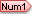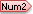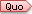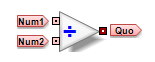← Back to X_TRADER® Help Library

### ADL® Documentation

#### Divide Block

Divide Block divides the first inputby the second inputand outputs the quotient.

Order of inputs affects the outcome.

Note: Dividing a zero by another zero will output a NaN (Not A Number). In a similar manner, dividing a non-zero number by a zero will output a positive or a negative Infinity. Keep in mind that these values are recognized by ADL as invalid inputs.

##### Operations